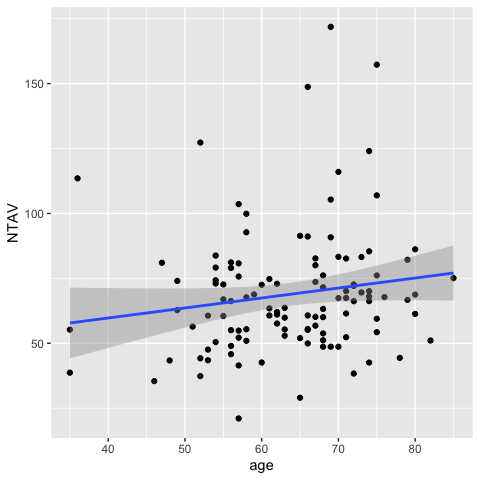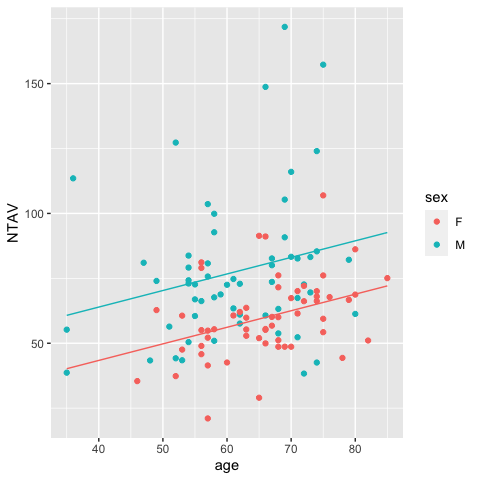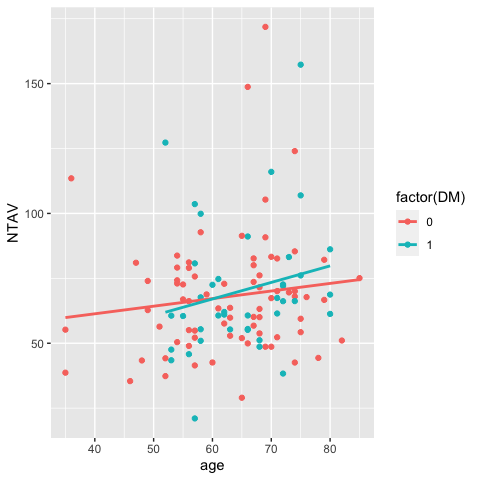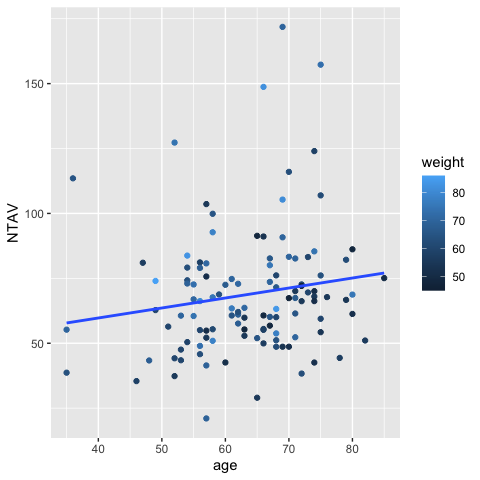# ggPredict() - Visualize multiple regression model

#### 2020-10-06

``````To reproduce this document, you have to install R package ggiraphExtra from github.
install.packages("devtools")
devtools::install_github("cardiomoon/ggiraphExtra")``````

# Linear regression Model

## Simple linear regression model

In univariate regression model, you can use scatter plot to visualize model. For example, you can make simple linear regression model with data `radial` included in package moonBook. The radial data contains demographic data and laboratory data of 115 patients performing IVUS(intravascular ultrasound) examination of a radial artery after tansradial coronary angiography. The NTAV(normalized total atheroma volume measured by intravascular ultrasound(IVUS) in cubic mm) is a quantitative measurement of atherosclerosis. Suppose you want to predict the amount of atherosclerosis(NTAV) from age.

``require(moonBook)   # for use of data radial``
``Loading required package: moonBook``
``````fit=lm(NTAV~age,data=radial)
summary(fit)``````
``````
Call:
lm(formula = NTAV ~ age, data = radial)

Residuals:
Min      1Q  Median      3Q     Max
-45.231 -14.626  -4.803   9.685 100.961

Coefficients:
Estimate Std. Error t value Pr(>|t|)
(Intercept)  44.3398    14.6251   3.032  0.00302 **
age           0.3848     0.2271   1.694  0.09302 .
---
Signif. codes:  0 '***' 0.001 '**' 0.01 '*' 0.05 '.' 0.1 ' ' 1

Residual standard error: 23.94 on 113 degrees of freedom
Multiple R-squared:  0.02477,   Adjusted R-squared:  0.01614
F-statistic:  2.87 on 1 and 113 DF,  p-value: 0.09302``````

You can get the regression equation from summary of regression model:

``y=0.38*x+44.34``

You can visualize this model easily with ggplot2 package.

``````require(ggplot2)
ggplot(radial,aes(y=NTAV,x=age))+geom_point()+geom_smooth(method="lm")``````You can make interactive plot easily with ggPredict() function included in ggiraphExtra package.

``````require(ggiraph)
require(ggiraphExtra)
require(plyr)
ggPredict(fit,se=TRUE,interactive=TRUE)``````

With this plot, you can identify the points and see the regression equation with your mouse.

## Multiple regression model without interaction

You can make a regression model with two predictor variables. Now you can use age and sex as predictor variables.

``````fit1=lm(NTAV~age+sex,data=radial)
summary(fit1)``````
``````
Call:
lm(formula = NTAV ~ age + sex, data = radial)

Residuals:
Min      1Q  Median      3Q     Max
-46.025 -12.687  -1.699   5.784  89.419

Coefficients:
Estimate Std. Error t value Pr(>|t|)
(Intercept)  17.8697    14.3846   1.242  0.21673
age           0.6379     0.2134   2.989  0.00344 **
sexM         20.5476     4.1943   4.899 3.27e-06 ***
---
Signif. codes:  0 '***' 0.001 '**' 0.01 '*' 0.05 '.' 0.1 ' ' 1

Residual standard error: 21.82 on 112 degrees of freedom
Multiple R-squared:  0.1969,    Adjusted R-squared:  0.1825
F-statistic: 13.73 on 2 and 112 DF,  p-value: 4.659e-06``````

From the result of regression analysis, you can get regression regression equations of female and male patients :

``````For female patient, y=0.64*x+17.87
For male patient, y=0.64*x+38.42``````

You can visualize this model with ggplot2 package.

``````equation1=function(x){coef(fit1)*x+coef(fit1)}
equation2=function(x){coef(fit1)*x+coef(fit1)+coef(fit1)}

ggplot(radial,aes(y=NTAV,x=age,color=sex))+geom_point()+
stat_function(fun=equation1,geom="line",color=scales::hue_pal()(2))+
stat_function(fun=equation2,geom="line",color=scales::hue_pal()(2))``````You can make interactive plot easily with ggPredict() function included in ggiraphExtra package.

``ggPredict(fit1,se=TRUE,interactive=TRUE)``

## Multiple regression model with interaction

You can make a regession model with two predictor variables with interaction. Now you can use age and DM(diabetes mellitus) and interaction between age and DM as predcitor variables.

``````fit2=lm(NTAV~age*DM,data=radial)
summary(fit2)``````
``````
Call:
lm(formula = NTAV ~ age * DM, data = radial)

Residuals:
Min      1Q  Median      3Q     Max
-44.094 -15.115  -4.093   9.102 102.024

Coefficients:
Estimate Std. Error t value Pr(>|t|)
(Intercept)  49.6463    16.8660   2.944  0.00395 **
age           0.2925     0.2648   1.105  0.27174
DM          -20.8618    34.8936  -0.598  0.55115
age:DM        0.3453     0.5353   0.645  0.52026
---
Signif. codes:  0 '***' 0.001 '**' 0.01 '*' 0.05 '.' 0.1 ' ' 1

Residual standard error: 24.1 on 111 degrees of freedom
Multiple R-squared:  0.0292,    Adjusted R-squared:  0.002966
F-statistic: 1.113 on 3 and 111 DF,  p-value: 0.347``````

The regression equation in this model are as follows: For patients without DM(DM=0), the intercept is 49.65 and the slope is 0.29. For patients with DM(DM=1), the intercept is 49.65-20.86 and the slope is 0.29+0.35.

``````For patients without DM(DM=0), y=0.29*x+49.65
For patients without DM(DM=1), y=0.64*x+28.78``````

You can visualize this model with ggplot2.

``ggplot(radial,aes(y=NTAV,x=age,color=factor(DM)))+geom_point()+stat_smooth(method="lm",se=FALSE)``You can make interactive plot easily with ggPredict() function included in ggiraphExtra package.

``ggPredict(fit2,colorAsFactor = TRUE,interactive=TRUE)``

## Multiple regression model with two continuous predictor variables with or without interaction

You can make a regession model with two continuous predictor variables. Now you can use age and weight(body weight in kilogram) as predcitor variables.

``````fit3=lm(NTAV~age*weight,data=radial)
summary(fit3)``````
``````
Call:
lm(formula = NTAV ~ age * weight, data = radial)

Residuals:
Min      1Q  Median      3Q     Max
-48.482 -13.815  -2.079   6.886  93.187

Coefficients:
Estimate Std. Error t value Pr(>|t|)
(Intercept)  37.60533  111.46748   0.337    0.736
age          -0.32698    1.69737  -0.193    0.848
weight       -0.10416    1.74620  -0.060    0.953
age:weight    0.01468    0.02687   0.546    0.586

Residual standard error: 22.91 on 111 degrees of freedom
Multiple R-squared:  0.1222,    Adjusted R-squared:  0.09851
F-statistic: 5.152 on 3 and 111 DF,  p-value: 0.00226``````

From the analysis, you can get the regression equation for a patient with body weight 40kg, the intercept is 37.61+(-0.10416)*40 and the slope is -0.33+0.01468*40

``````For bodyweight 40kg, y=0.26*x+33.44
For bodyweight 50kg, y=0.41*x+32.4
For bodyweight 90kg, y=0.99*x+28.23``````

To visualize this model, the simple ggplot command shows only one regression line.

``ggplot(radial,aes(y=NTAV,x=age,color=weight))+geom_point()+stat_smooth(method="lm",se=FALSE)``You can easily show this model with ggPredict() function.

``ggPredict(fit3,interactive=TRUE)``

### Multiple regression model with three predictor variables

You can make a regession model with three predictor variables. Now you can use age and weight(body weight in kilogram) and HBP(hypertension) as predcitor variables.

``````fit4=lm(NTAV~age*weight*HBP,data=radial)
summary(fit4)``````
``````
Call:
lm(formula = NTAV ~ age * weight * HBP, data = radial)

Residuals:
Min      1Q  Median      3Q     Max
-43.453 -14.125  -3.226   7.724  88.126

Coefficients:
Estimate Std. Error t value Pr(>|t|)
(Intercept)      64.11678  155.82328   0.411    0.682
age              -0.67650    2.47339  -0.274    0.785
weight           -0.39685    2.37886  -0.167    0.868
HBP            -101.94261  238.52253  -0.427    0.670
age:weight        0.01686    0.03804   0.443    0.658
age:HBP           1.27972    3.64467   0.351    0.726
weight:HBP        1.52494    3.75529   0.406    0.685
age:weight:HBP   -0.01666    0.05777  -0.288    0.774

Residual standard error: 22.8 on 107 degrees of freedom
Multiple R-squared:  0.1626,    Adjusted R-squared:  0.1078
F-statistic: 2.967 on 7 and 107 DF,  p-value: 0.006982``````

From the analysis result, you can get the regression equation for a patient without hypertension(HBP=0) and body weight 60kg: the intercept is 64.12+(-0.39685*60) and the slope is -0.67650+(0.01686*60). The equation for a patient with hypertension(HBP=1) and same body weight: the intercept is 64.12+(-0.39685*60-101.94) and the slope is -0.67650+(0.01686*60)+1.27972+(-001666*60).

To visualize this model, you can make a faceted plot with ggPredict() function. You can see the regression equation of each subset with hovering your mouse on the regression lines.

``ggPredict(fit4,interactive = TRUE)``

# Logistic regression model

## Multiple logistic regression model with two predictor variables

### Model with interaction

You can use glm() function to make a logistic regression model. The GBSG2 data in package TH.data contains data from German Breast Cancer Study Group 2. Suppose you want to predict survival with number of positive nodes and hormonal therapy.

``````require(TH.data) # for use data GBSG2
fit5=glm(cens~pnodes*horTh,data=GBSG2,family=binomial)
summary(fit5)``````
``````
Call:
glm(formula = cens ~ pnodes * horTh, family = binomial, data = GBSG2)

Deviance Residuals:
Min       1Q   Median       3Q      Max
-2.7892  -1.0208  -0.7573   1.2288   1.6667

Coefficients:
Estimate Std. Error z value Pr(>|z|)
(Intercept)     -0.55368    0.13942  -3.971 7.15e-05 ***
pnodes           0.08672    0.02172   3.993 6.53e-05 ***
horThyes        -0.69833    0.25394  -2.750  0.00596 **
pnodes:horThyes  0.06306    0.03899   1.617  0.10582
---
Signif. codes:  0 '***' 0.001 '**' 0.01 '*' 0.05 '.' 0.1 ' ' 1

(Dispersion parameter for binomial family taken to be 1)

Null deviance: 939.68  on 685  degrees of freedom
Residual deviance: 887.69  on 682  degrees of freedom
AIC: 895.69

Number of Fisher Scoring iterations: 4``````

You can easily visualize this model with ggPredict() function.

``ggPredict(fit5,se=TRUE,interactive=TRUE,digits=3)``
``````## Warning in eval(family\$initialize): non-integer #successes in a binomial glm!

## Warning in eval(family\$initialize): non-integer #successes in a binomial glm!``````